最小二乘法
https://en.wikipedia.org/wiki/Matrix_calculus
接下来，对$\boldsymbol{\omega}$求导：
$\large\boldsymbol{\frac{\partial{RSS}}{\partial{\omega}}=\frac{\partial{||y-X\omega||_2\,^2}}{\partial{\omega}}=\frac{\partial{(y-X\omega)^T(y-X\omega)}}{\partial{\omega}}}$
$\because(A-B)^T=A^T-B^T并且(AB)^T=B^T*A^T\\\therefore=\large\boldsymbol{\frac{\partial{(y^T-\omega^TX^T)(y-X\omega)}}{\partial{\omega}}=\frac{\partial{(y^Ty-\omega^TX^Ty-y^TX\omega+\omega^TX^TX\omega)}}{\partial{\omega}}}$
$\because$ 矩阵求导中，α为常数，有如下规则：
$\large\boldsymbol{\frac{\partial\alpha}{\partial{A}}=0,\frac{\partial{A^TB^TC}}{\partial{A}}=B^TC,\frac{\partial{C^TBA}}{\partial{A}}=B^TC,\frac{\partial{A^TBA}}{\partial{A}}=(B+B^T)A}$
$\large\boldsymbol{=0-X^Ty-X^Ty+2X^TX\omega}\\\large\boldsymbol{=X^TX\omega-X^Ty}$
我们让求导后的一阶导数为0：
$\large\boldsymbol{X^TX\omega-X^Ty}=0$
$\large\boldsymbol{X^TX\omega=X^Ty}$
左乘一个$\large\boldsymbol{(X^TX)^{-1}}$则有：
$\large\boldsymbol{\omega=(X^TX)^{-1}X^Ty}$
到了这里，希望能够将$\boldsymbol{\omega}$留在等式的左边，其他与特征矩阵有关的部分都放到等式的右边，如此就可以求出$\boldsymbol{\omega}$的最优解了。这个功能非常容易实现，只需要我们左乘$\boldsymbol{X^TX}$的逆矩阵就可以。在这里，逆矩阵存在的充分必要条件是特征矩阵不存在多重共线性。
假设矩阵的逆是存在的，此时$\boldsymbol{\omega}$就是我们参数的最优解。求出这个参数向量，就解出了$\boldsymbol{X\omega}$，也就能够计算出预测值$\boldsymbol{\hat{y}}$了。对于多元线性回归的理解，到这里就足够了，如果还希望继续深入，可以给一个方向：矩阵$\boldsymbol{X^TX}$其实是使用奇异值分解来进行求解的，可以仔细去研究一下。以算法工程师和数据挖掘工程师为目标的人，能够手动推导上面的求解过程是基本要求。
除了多元线性回归的推导之外，这里还需要提到一些在上面的推导过程中不曾被体现出来的问题。在统计学中，使用最小二乘法来求解线性回归的方法是一种“无偏估计”的方法，这种无偏估计要求因变量，也就是标签的分布必须服从正态分布。这是说，y必须经由正太化处理（比如说取对数，或者使用QuantileTransformer或者PowerTransformer这些功能）。在机器学习中，会先考虑模型的效果，如果模型效果不好，那可能考虑改变因变量的分布。


展开全文机器学习 算法
• 基于jupyter notebook的python编程—–利用梯度下降算法求解多元线性回归方程，并与最小二乘法求解进行精度对比目录一、梯度下降算法的基本原理1、梯度下降算法的基本原理二、题目、表格数据、以及python环境搭建1、...
• 各位大神，小弟刚学VC++，想通过一组大量的数据来计算某个公式的系数，类似于：Q=aX+bY+cZ不知道该如何用vc来编写程序，用来计算abc三个待定系数的值，望告之，谢谢。
• 利用梯度下降算法求解多元线性回归方程，并与最小二乘法求解进行精度对比 基于jupyter notebook的python编程 这里写目录标题利用梯度下降算法求解多元线性回归方程，并与最小二乘法求解进行精度对比基于jupyter ...
基于jupyter notebook的python编程
利用梯度下降算法求解多元线性回归方程，并与最小二乘法求解进行精度对比基于jupyter notebook的python编程0.问题描述1.  导入库、数据、并为变量赋值2.定义系数初始值以及学习率和迭代次数3.定义最小二乘法函数-损失函数（代价函数）4.定义梯度下降算法求解线性回归方程系数python函数5.代用函数，进行系数求解，并打印6.画出回归方程线性拟合图7.运行结果最小二乘法法求解多元线性回归方程的python代码实现1.导入库，并为变量赋值2.创建系数矩阵，并求解系数矩阵W，截距b、a1、和a23.画出线性回归图4.求解出各项值5.运行结果总结对比
0.问题描述
多元线性回归问题描述如下：
为求得某个地区的商品店的月营业额是与店铺的面积相关性大，还是与该店距离车站距离的相关性大，需要我们以店铺面积、距离车站的距离、以及月营业额建立线性回归方程，并求解该方程，和相关系数：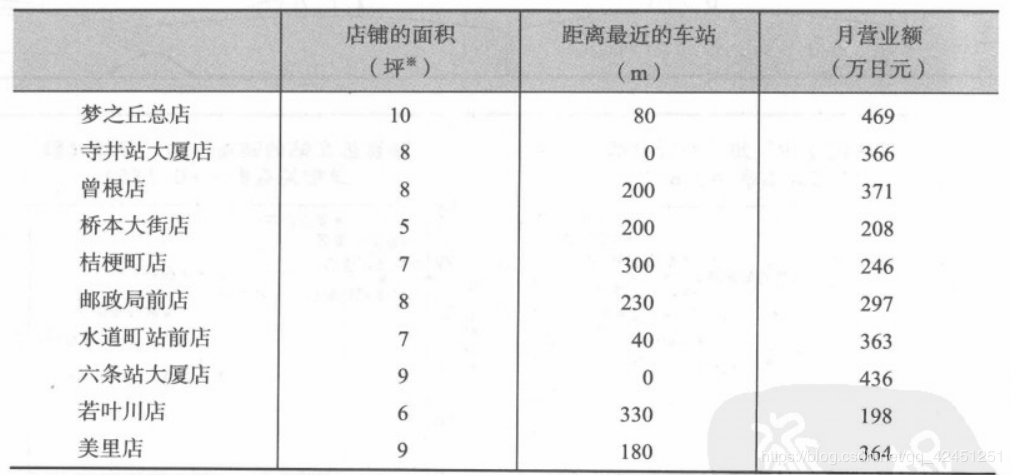所用的数据
自左到右依次代表店铺面积、距离车站距离和月营业额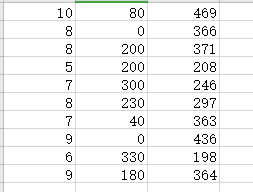1.  导入库、数据、并为变量赋值
import numpy as np
from matplotlib import pyplot as plt
from mpl_toolkits.mplot3d import Axes3D
data=np.genfromtxt('D:/面积距离车站数据.csv',delimiter=',')
x_data=data[:,:-1]
y_data=data[:,2]


2.定义系数初始值以及学习率和迭代次数
#定义学习率、斜率、截据
#设方程为y=theta1*x1+theta2*x2+theta0
lr=0.00001
theta0=0
theta1=0
theta2=0
#定义最大迭代次数，因为梯度下降法是在不断迭代更新k与b
epochs=10000


3.定义最小二乘法函数-损失函数（代价函数）
#定义最小二乘法函数-损失函数（代价函数）
def compute_error(theta0,theta1,theta2,x_data,y_data):
totalerror=0
for i in range(0,len(x_data)):#定义一共有多少样本点
totalerror=totalerror+(y_data[i]-(theta1*x_data[i,0]+theta2*x_data[i,1]+theta0))**2


4.定义梯度下降算法求解线性回归方程系数python函数
#梯度下降算法求解参数
m=len(x_data)
for i in range(epochs):
for j in range(0,m):
return theta0,theta1,theta2


5.代用函数，进行系数求解，并打印
#进行迭代求解
print('结果：迭代次数：{0} 学习率：{1}之后 a0={2},a1={3},a2={4},代价函数为{5}'.format(epochs,lr,theta0,theta1,theta2,compute_error(theta0,theta1,theta2,x_data,y_data)))
print("多元线性回归方程为:y=",theta1,"X1+",theta2,"X2+",theta0)


6.画出回归方程线性拟合图
#画图
ax.scatter(x_data[:,0],x_data[:,1],y_data,c='r',marker='o')
x0=x_data[:,0]
x1=x_data[:,1]
#生成网格矩阵
x0,x1=np.meshgrid(x0,x1)
z=theta0+theta1*x0+theta2*x1
#画3d图
ax.plot_surface(x0,x1,z)
ax.set_xlabel('area')
ax.set_ylabel('distance')
ax.set_zlabel("Monthly turnover")
plt.show()


7.运行结果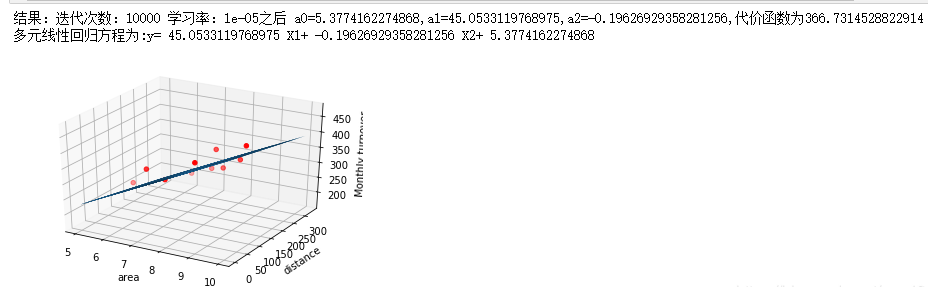最小二乘法法求解多元线性回归方程的python代码实现
与梯度下降算法作对比基于jupyter notebook的python编程0.问题描述1.  导入库、数据、并为变量赋值2.定义系数初始值以及学习率和迭代次数3.定义最小二乘法函数-损失函数（代价函数）4.定义梯度下降算法求解线性回归方程系数python函数5.代用函数，进行系数求解，并打印6.画出回归方程线性拟合图7.运行结果最小二乘法法求解多元线性回归方程的python代码实现1.导入库，并为变量赋值2.创建系数矩阵，并求解系数矩阵W，截距b、a1、和a23.画出线性回归图4.求解出各项值5.运行结果总结对比
1.导入库，并为变量赋值
import numpy as np
import matplotlib.pyplot as plt
import seaborn as sns
import pandas as pd
%matplotlib inline
data = np.genfromtxt("D:/面积距离车站数据.csv",delimiter=",")
X1=data[0:10,0]#自变量温度
X2=data[0:10,1]#因变量销售量
Y=data[0:10,2]#自变量温度
#将因变量赋值给矩阵Y1
Y1=np.array([Y]).T
#为自变量系数矩阵X赋值
X11=np.array([X1]).T
X22=np.array([X2]).T

2.创建系数矩阵，并求解系数矩阵W，截距b、a1、和a2
A=np.array([,,,,,,,,,])#创建系数矩阵
B=np.hstack((A,X11))#将矩阵a与矩阵X11合并为矩阵b
X=np.hstack((B,X22))#将矩阵b与矩阵X22合并为矩阵X
#求矩阵X的转置矩阵
X_=X.T
#求矩阵X与他的转置矩阵的X_的乘积
X_X=np.dot(X_,X)
#求矩阵X与他的转置矩阵的X_的乘积的逆矩阵
X_X_=np.linalg.inv(X_X)
#求解系数矩阵W，分别对应截距b、a1、和a2
W=np.dot(np.dot((X_X_),(X_)),Y1)
b=W
a1=W
a2=W
print("系数a1=",a1)
print("系数a2=",a2)
print("截距为=",b)
print("多元线性回归方程为:y=",a1,"X1+",a2,"X2+",b)

3.画出线性回归图
#画出线性回归分析图
sns.pairplot(data1, x_vars=['area','distance'], y_vars='Y', height=3, aspect=0.8, kind='reg')
plt.show()

4.求解出各项值
#求月销售量Y的和以及平均值y1
sumy=0#因变量的和
y1=0#因变量的平均值
for i in range(0,len(Y)):
sumy=sumy+Y[i]
y1=sumy/len(Y)
#求月销售额y-他的平均值的和
y_y1=0#y-y1的值的和
for i in range(0,len(Y)):
y_y1=y_y1+(Y[i]-y1)
print("销售量-销售量平均值的和为:",y_y1)
#求预测值sales1
sales1=[]
for i in range(0,len(Y)):
sales1.append(a1*X1[i]+a2*X2[i]+b)
#求预测值的平均值y2
y2=0
sumy2=0
for i in range(len(sales1)):
sumy2=sumy2+sales1[i]
y2=sumy2/len(sales1)
#求预测值-平均值的和y11_y2
y11_y2=0
for i in range(0,len(sales1)):
y11_y2=y11_y2+(sales1[i]-y2)
print("预测销售值-预测销售平均值的和为:",y11_y2)
#求月销售额y-他的平均值的平方和
Syy=0#y-y1的值的平方和
for i in range(0,len(Y)):
Syy=Syy+((Y[i]-y1)*(Y[i]-y1))
print("Syy=",Syy)
#求y1-y1平均的平方和
Sy1y1=0
for i in range(0,len(sales1)):
Sy1y1=Sy1y1+((sales1[i]-y2)*(sales1[i]-y2))
print("Sy1y1=",Sy1y1)
#（y1-y1平均）*（y-y平均）
Syy1=0
for i in range(0,len(sales1)):
Syy1=Syy1+((Y[i]-y1)*(sales1[i]-y2))
print("Syy1=",Syy1)
#求R
R=Syy1/((Syy*Sy1y1)**0.5)
R2=R*R
print("判定系数R2=",R2)

5.运行结果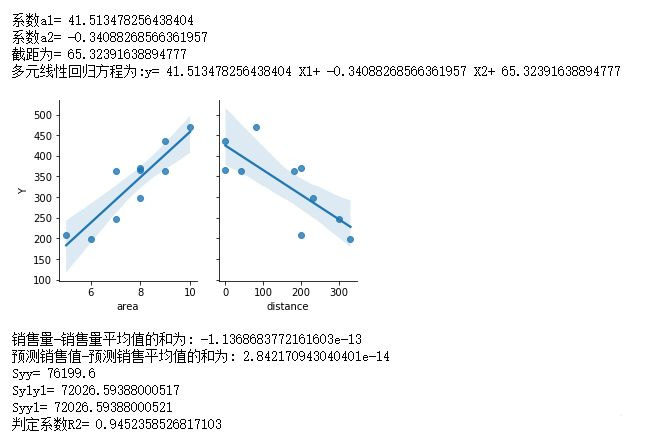总结对比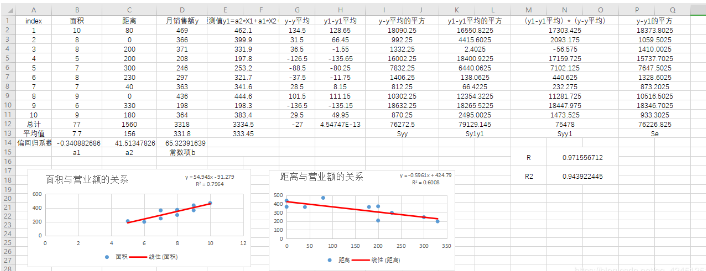通过以上对比结果可以知道，最小二乘法求解线性回归方程的精度优于梯度下降算法，这也是梯度下降算法的确定，求解精度太低，求解还需要很多次的迭代，所以，现代的人工智能机器学习的深度算法基本已经放弃梯度下降算法的求解，需要多次计算，这对计算机资源来说是一种浪费哦！现代多用于矩阵的最小二乘法进行线性回归系数的求解！


展开全文机器学习
• 通过为 lsqr 提供用来计算 A*x 和 A'*x 的函数句柄(而非系数矩阵 A)来求解线性方程组。创建一个非对称三对角矩阵。预览该矩阵。A = gallery('wilk',21) + diag(ones(20,1),1)A = 21×2110 2 0 0 0 0 0 0 0 0 0 0 0 ....
通过为 lsqr 提供用来计算 A*x 和 A'*x 的函数句柄(而非系数矩阵 A)来求解线性方程组。创建一个非对称三对角矩阵。预览该矩阵。A = gallery('wilk',21) + diag(ones(20,1),1)A = 21×2110     2     0     0     0     0     0     0     0     0     0     0     0     0     0     0     0     0     0     0     01     9     2     0     0     0     0     0     0     0     0     0     0     0     0     0     0     0     0     0     00     1     8     2     0     0     0     0     0     0     0     0     0     0     0     0     0     0     0     0     00     0     1     7     2     0     0     0     0     0     0     0     0     0     0     0     0     0     0     0     00     0     0     1     6     2     0     0     0     0     0     0     0     0     0     0     0     0     0     0     00     0     0     0     1     5     2     0     0     0     0     0     0     0     0     0     0     0     0     0     00     0     0     0     0     1     4     2     0     0     0     0     0     0     0     0     0     0     0     0     00     0     0     0     0     0     1     3     2     0     0     0     0     0     0     0     0     0     0     0     00     0     0     0     0     0     0     1     2     2     0     0     0     0     0     0     0     0     0     0     00     0     0     0     0     0     0     0     1     1     2     0     0     0     0     0     0     0     0     0     0⋮由于此三对角矩阵有特殊的结构，您可以用函数句柄来表示 A*x 运算。当 A 乘以向量时，所得向量中的大多数元素为零。结果中的非零元素对应于 A 的非零三对角元素。表达式 Ax 变为：Ax=[1020⋯⋯01920⋮01⋱20⋮010⋱⋱⋮0⋱1⋱0⋮⋱⋱⋱20⋯⋯0110][x1x2x3⋮⋮x21]=[10x1+2x2x1+9x2+2x3⋮⋮x19+9x20+2x21x20+10x21]。结果向量可以写为三个向量的和：Ax=[10x1+2x2x1+9x2+2x3⋮⋮x19+9x20+2x21x20+10x21]=[0x1x2⋮x20]+[10x19x2⋮9x2010x21]+2⋅[x2x3⋮x210]。同样，ATx 的表达式变为：ATx=[1010⋯⋯02910⋮02⋱10⋮020⋱⋱⋮0⋱1⋱0⋮⋱⋱⋱10⋯⋯0210][x1x2x3⋮⋮x21]=[10x1+x22x1+9x2+x3⋮⋮2x19+9x20+x212x20+10x21]。ATx=[10x1+x22x1+9x2+x3⋮⋮2x19+9x20+x212x20+10x21]=2⋅[0x1x2⋮x20]+[10x19x2⋮9x2010x21]+[x2x3⋮x210]。在 MATLAB® 中，编写一个函数来创建这些向量并将它们相加，从而根据标志输入给出 A*x 或 A'*x 的值：function y = afun(x,flag)if strcmp(flag,'notransp') % Compute A*xy = [0; x(1:20)] ...+ [(10:-1:0)'; (1:10)'].*x ...+ 2*[x(2:end); 0];elseif strcmp(flag,'transp') % Compute A'*xy = 2*[0; x(1:20)] ...+ [(10:-1:0)'; (1:10)'].*x ...+ [x(2:end); 0];endend(该函数作为局部函数保存在示例的末尾。)现在，通过为 lsqr 提供用于计算 A*x 和 A'*x 的函数句柄，求解线性方程组 Ax=b。使用容差 1e-6 和 25 次迭代。指定 b 为 A 的行总和，使得 x 的实际解是由 1 组成的向量。b = full(sum(A,2));tol = 1e-6;maxit = 25;x1 = lsqr(@afun,b,tol,maxit)lsqr converged at iteration 21 to a solution with relative residual 5.4e-13.x1 = 21×11.00001.00001.00001.00001.00001.00001.00001.00001.00001.0000⋮局部函数function y = afun(x,flag)if strcmp(flag,'notransp') % Compute A*xy = [0; x(1:20)] ...+ [(10:-1:0)'; (1:10)'].*x ...+ 2*[x(2:end); 0];elseif strcmp(flag,'transp') % Compute A'*xy = 2*[0; x(1:20)] ...+ [(10:-1:0)'; (1:10)'].*x ...+ [x(2:end); 0];endend
展开全文• 利用labview制作的简单最小二乘法求解线性方程工具 简单易操作 纯软件制作
• 机器学习线性回归模型 线性回归（linear regression）是一种线性模型，它假设输入变量 x 和单个输出变量 y 之间存在线性关系 具体来说，利用线性回归模型，可以从一组输入变量 x 的线性组合中，计算输出变量 y ...
机器学习线性回归模型
线性回归（linear regression）是一种线性模型，它假设输入变量 x 和单个输出变量 y 之间存在线性关系
具体来说，利用线性回归模型，可以从一组输入变量 x 的线性组合中，计算输出变量 y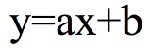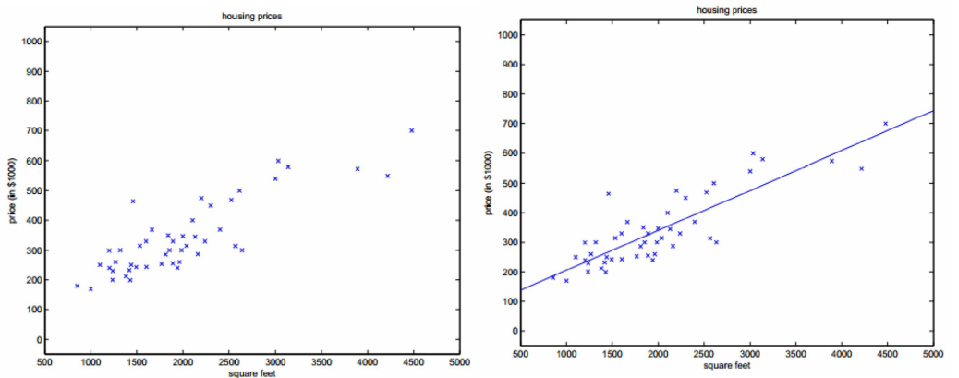给定有d个属性（特征）描述的示例 x =（x1; x2; …; xd），其中xi是x在第i个属性（特征）上的取值，线性模型（linear model）试图学得一个通过属性（特征）的线性组合来进行预测的函数，即：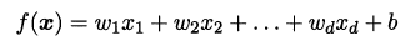一般用向量形式写成：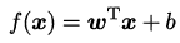其中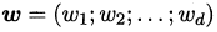假设特征和结果都满足线性，即不大于一次方。
w和b学得之后，模型就得以确定。

许多功能更为强大的非线性模型可在线性模型的基础上通过引入层级结构或高维映射而得。
最小二乘法求解
基于均方误差最小化来进行模型求解的方法称为“最小二乘法”（least square method）
它的主要思想就是选择未知参数，使得理论值与观测值之差的平方和达到最小。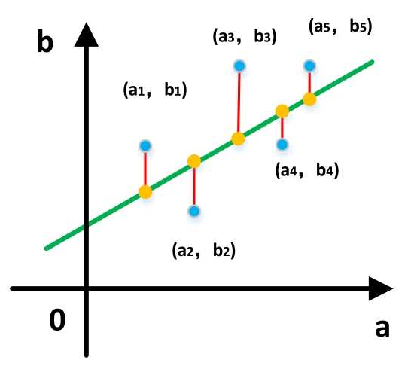我们假设输入属性（特征）的数目只有一个：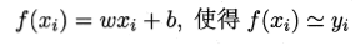在线性回归中，最小二乘法就是试图找到一条直线，使所有样本到直线上的欧式距离之和最小。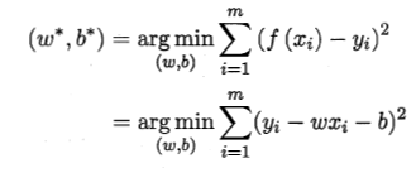求解线性回归
求解w和b，使得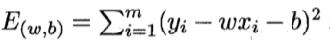最小化的过程，称为线性回归模型的“最小二乘参数估计”
将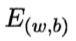分别对w和b求导，可以得到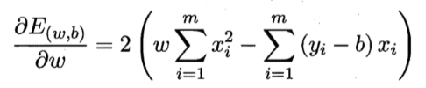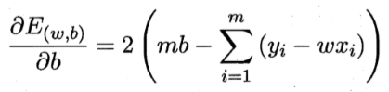令偏导数都为0，可以得到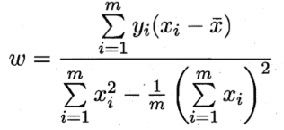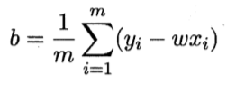—— 其中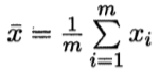代码实现
### 0.引入依赖
import numpy as np
import matplotlib.pyplot as plt

### 1.导入数据
points = np.genfromtxt('data.csv',delimiter=',')

points[0,0]

# 提取points中的两列数据，分别作为x,y
x = points[:, 0]
y = points[:, 1]

# 用plt画出散点图
plt.scatter(x, y)
plt.show()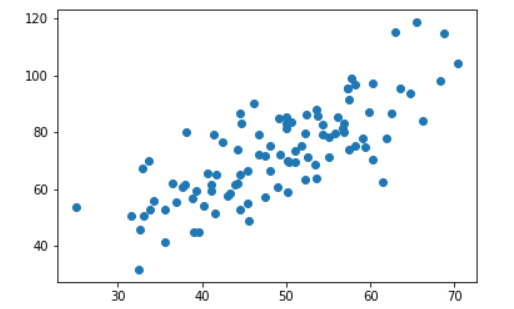###  2.定义损失函数
# 损失函数是系数的函数，另外还要传入数据的x，y
def compute_cost(w, b, points):
total_cost = 0
M = len(points)

#逐点计算平方损失，然后计算平均值
for i in range(M):
x = points[i, 0]
y = points[i, 1]
total_cost += (y - w*x -b) ** 2

### 3.定义算法拟合函数
# 先定义一个求均值的函数
def average(data):
sum = 0
num = len(data)
for i in range(num):
sum += data[i]

return sum/num

# 定义核心拟合函数
def fit(points):
M = len(points)
x_bar = average(points[:,0])

sum_yx = 0
sum_x2 = 0
sum_delta = 0

for i in range(M):
x = points[i, 0]
y = points[i, 1]
sum_yx += y * (x - x_bar)
sum_x2 += x ** 2
# 根据公式计算w
w = sum_yx / (sum_x2 - M * (x_bar ** 2))

for i in range(M):
x = points[i, 0]
y = points[i, 1]
sum_delta += (y - w * x)
b = sum_delta / M

return w, b

### 4. 测试
w ,b = fit(points)

print("w is: ", w)
print("b is: ", b)

cost = compute_cost(w, b, points)

print("cost is : ",cost)

### 5.画出拟合曲线
plt.scatter(x,y)

#针对每一个x，计算得出预测的y值
pred_y = w * x + b

plt.plot(x, pred_y, c='r')
plt.show()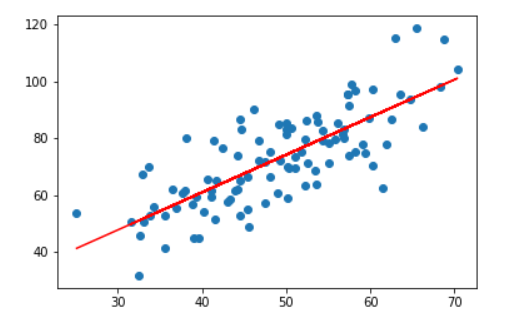展开全文算法 机器学习 python
• 使用Python编程，分别根据梯度法和最小二乘法求解多元函数问题分别使用梯度下降法和最小二乘法求解多元函数并进行比较，这里使用jupyter notebook平台进行Python编程一、题目描述二、使用梯度下降法求解多元函数（一...机器学习python 人工智能 机器学习 梯度下降算法
• jupyter用最小二乘法求解多元线性回归方程jupyter用最小二乘法求解多元线性回归方程导入需要的数据文件编写程序遇到的问题及解决方法 导入需要的数据文件 首先将所需要的数据文件导入到jupyter中，就可以不用在程序...人工智能 python
• 引言最小二乘法是经典的参数稳健估计方法。核心思想是使得估计出的模型与实际数据之间误差的平方和最小（趋于+∞\infty）。直线参数的估计又是最常用的曲线参数估计，稳健估计方法有很多：RANSAC、BaySAC、极大似然...
• 所谓线性回归方程其实就是最简单的一元方程,而我们需要做的就是找到那个最好的斜率使得这条线离所有的点的距离之和最小.用数学的说法是使所有点到直线的距离的均方差最小. 上面是一组散点,我们想找到一条直线最契合...
• 多元线性回归最小二乘法及其经济分析[J]. 经济师,2003,11:129. 还可以采用最小二乘法来估计参数： 算法设计也可以参考两种系数最终公式设计。 下面的Java代码由网友设计，采用第一种方法...
• 把非线性转化为线性，再求解。在制定公差标准时，必须掌握加工的极限误差随工件尺寸变化的规律。例如，对用普通车床切削外圆进行了大量实验，得到加工极限误差t与工作直径D的统计资料...再根据一元回归最小二乘法计算：MATLAB
• 前面分析了 线性最小二乘 的解法YcoFlegs：[数值计算] 数据拟合——线性最小二乘法​zhuanlan.zhihu.com现在来看另一个问题：非线性最小二乘法1. 定义首先是如何定义这里这个“非线性”。为什么前面用多项式拟合就是...
• 1拟合h(x) = w0 + w1*x话不多说，直接上code1.1代码# -*- coding: utf-8 -*- ...Aim：最小二乘法库函数leastsq使用示例详解 """ import numpy as np import matplotlib.pyplot as plt from ......